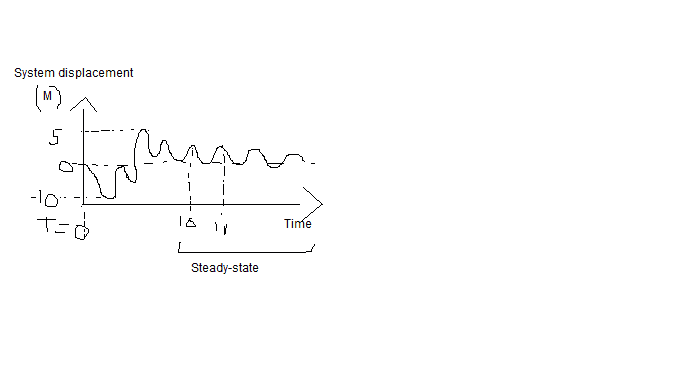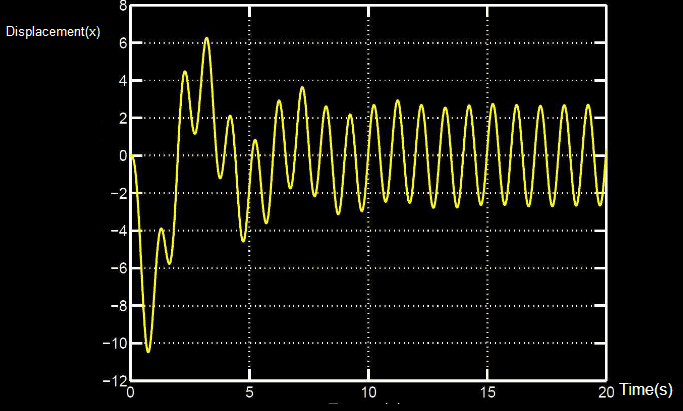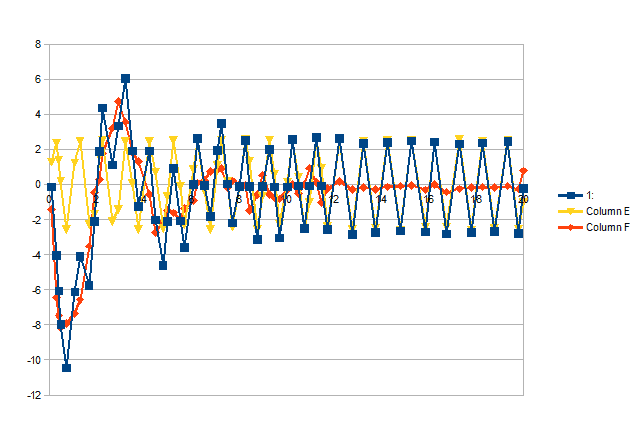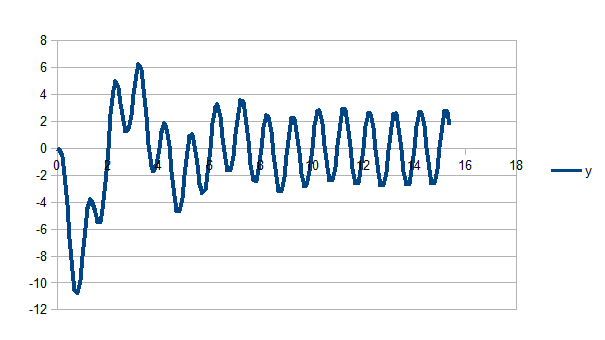# Measuring the natural frequency of a spring-mass system driving force

EpselonZero
Homework Statement:
Measuring the natural frequency of a spring-mass system with the driving force graph
Relevant Equations:
$$F = \frac{\omega}{2\pi}, \omega = \frac{2\pi}{T}$$
Hi,

On a driving force graph ##y = displacement (m)## and ##x = time## where the external force start at t = 0 and the system is in equilibrium at x=0, it's easy to find the driving frequency.

$$F = \frac{\omega}{2\pi}, \omega = \frac{2\pi}{T}$$ and we can get ##T## easily with the steady state part of the graph.

However, is there a way to find the natural frequency and the driving force amplitude?

Maybe by finding where ##w_d = w_0##, which is the resonance frequency.Last edited:

## Answers and Replies

Homework Helper
Gold Member
The natural frequency can be inferred from the phase lag. To find that, you need the corresponding graph for the applied force.

EpselonZero
The natural frequency can be inferred from the phase lag. To find that, you need the corresponding graph for the applied force.
I have to find the natural frequency, but that's all I have. Is there another way?

Should I use ##F(t) = F_0=cos(\omega t + \phi)##

Homework Helper
Gold Member
I have to find the natural frequency, but that's all I have. Is there another way?

Should I use ##F(t) = F_0=cos(\omega t + \phi)##
What exactly do you have? The graph in post #1 looks like a freehand sketch. How was this obtained?
The start of it shows some transients. It might be possible to estimate a natural frequency from that, but it looks a bit messy.

EpselonZero
It might be possible to estimate a natural frequency from that, but it looks a bit messy.
I'll try to draw a better graph. I'll edited the post.

Homework Helper
Gold Member
I'll try to draw a better graph.
But from what? Do you have some data you can post (in machine-readable form, not as an image)?

EpselonZero
But from what? Do you have some data you can post (in machine-readable form, not as an image)?
I have to find the natural frequency only with that graph. I don't have any data.

Homework Helper
Gold Member
I have to find the natural frequency only with that graph. I don't have any data.
But if you are drawing the graph, what are you using to draw it?

EpselonZero
I try to draw the same graph that I have in my book with my mouseHomework Helper
Gold Member
I try to draw the same graph that I have in my book with my mouseCan you simply photograph the page in the book and post that?

EpselonZeroIs it good?

Homework Helper
Gold Member
Yes, much better.
The general solution to forced damped oscillation, in complex form, is ##z(t)=Ce^{-\alpha t}+De^{-\alpha' t}+(A+iB)e^{-i\omega_dt}##, where ##\omega_d## is the forcing angular frequency, α and α are determined by the natural frequency and the damping factor, and A and B are determined by the forcing amplitude and frequency, the mass, the damping factor ##\Gamma## and the natural frequency ##\omega_0##.
E.g. ##A=\frac{(\omega_0^2-\omega_d^2)\frac{F_0}m}{(\omega_0^2-\omega_d^2)+\Gamma^2\omega_d^2}##.
See https://ocw.mit.edu/courses/physics...-fall-2016/syllabus/MIT8_03SCF16_Text_Ch2.pdf, but I have used the opposite sign for alpha.

The ##Ce^{-\alpha t}+De^{-\alpha' t}## terms are transients and die away, so the other terms correspond to the last part of your graph. In the early part of your graph you have the same but with the transient added in. So if you extend that trailing part back to the start and subtract it from the whole you should be left with a graph of the transients only.

Last edited:
EpselonZero
The ##Ce^{-\alpha t}+De^{-\alpha' t}## terms are transients and die away, so the other terms correspond to the last part of your graph. In the early part of your graph you have the same but with the transient added in. So if you extend that trailing part back to the start and subtract it from the whole you should be left with a graph of the transients only.

I understand that, but how I can subtract the ##\omega_d## from the transients with only the data that I have. Which are, ##Mass = 1##, at ## x = 0## the mass doesn't move. ##F(t) = f_0cos(\omega t + \phi)## and the graph.

I found ##\omega_d## using the graph, but I don't have the amplitude, ##F_0## or ##\gamma## which is ##\frac{b}{m}##

Basically, I have to find all those values above.

Last edited:
Homework Helper
Gold Member
I understand that, but how I can subtract the ##\omega_d## from the transients with only the data that I have. Which are, ##Mass = 1##, at ## x = 0## the mass doesn't move. ##F(t) = f_0cos(\omega t + \phi)## and the graph.

I found ##\omega_d## using the graph, but I don't have the amplitude, ##F_0## or ##\gamma## which is ##\frac{b}{m}##

Basically, I have to find all those values above.
I mean to subtract the displacements as shown on the graph. That should produce a graph of ##Ce^{-\alpha t}##, allowing you to read off C and ##\alpha##.
Of course, it would be a lot easier if you had the raw data instead of just a picture.

EpselonZero
Of course, it would be a lot easier if you had the raw data instead of just a picture.

I have multiple graphs and I have to read those data only with the graph.

The order of the questions is the same. I mean, I have to find ##\omega_d## then ##\omega_0##, ##b## , ##F_0## and ##\phi##

So, I guess I need ##\omega_d## to get ##\omega_0## and so on.

Maybe I can only get ##\omega_d## with the graph then I have to use ##\omega_d## to get ##\omega_0##, but even after hours I don't see how.

Homework Helper
Gold Member
I have multiple graphs and I have to read those data only with the graph.

The order of the questions is the same. I mean, I have to find ##\omega_d## then ##\omega_0##, ##b## , ##F_0## and ##\phi##

So, I guess I need ##\omega_d## to get ##\omega_0## and so on.

Maybe I can only get ##\omega_d## with the graph then I have to use ##\omega_d## to get ##\omega_0##, but even after hours I don't see how.
You have ##\omega_d## already, yes? I've just downloaded a tool for extracting datapoints from a graph image. I'll let you know how I go.

EpselonZero
You have ##\omega_d## already, yes?

##\omega_d = \frac{2\pi}{T}##

We can see in the steady-state that the period = 1s, so ##\omega_d = 2\pi## and ##f = 1hz##

In the steady-state, the oscillator moves with the same angular frequency as the drive.

Homework Helper
Gold MemberHere's what I got. The blue is a digitisation of points on your graph. The yellow is points of a sine curve based on the trailing part of your graph, and you see that it matches the blue well there.
The red is obtained by subtracting yellow from blue. This reveals the transients that die away. It's a bit scrappy because my digitisation wasn't that great, but you can see the 4 second cycle (underdamped) and you can estimate the attenuation per cycle.
Can you make some progress from there?

EpselonZero
So ##\omega_0 = \frac{2\pi}{4}## Basically, the transients is always (in this case) blue - yellow?

Homework Helper
Gold Member
So ##\omega_0 = \frac{2\pi}{4}##
Not so fast. This is an underdamped oscillation. The relationship between the observed period of the transient (ω) and ω0 is given by equation 2.9 at the link I posted. So you need to find Γ. You can find that from the observed attenuation of the transient and equation 2.10.
the transients is always (in this case) blue - yellow?
It is always the ##Ce^{-\alpha t}## term in ##z(t)=Ce^{-\alpha t}+De^{-\alpha' t}+(A+iB)e^{-i\omega_dt}##. The steady state behaviour, after transients have died away, is given by the other terms. So if you extrapolate the steady state back to the origin and subtract it from the whole graph you are left with the graph of the transients.

EpselonZero
How can I see this is a underdamped oscillator and the period without your graph.

Gold Member
The graph you posted in post 11 makes it easy to visually find the natural frequency.

Look at the graph around the 10sec mark where it crosses the -2 displacement going in the positive direction. Then count how many times the graph crosses the -2 displacement, in the same direction, between the 10sec and 20sec marks.

Thus knowing how many cycles in 10sec, can you determine the frequency?

Cheers,
Tom

EpselonZero
Look at the graph around the 10sec mark where it crosses the -2 displacement going in the positive direction. Then count how many times the graph crosses the -2 displacement, in the same direction, between the 10sec and 20sec marks.

Between 10s and 20s isn't ##\omega_d##? Since in the steady-state, the oscillator moves with the same angular frequency as the drive.

Homework Helper
Gold Member
The graph you posted in post 11 makes it easy to visually find the natural frequency.

Look at the graph around the 10sec mark where it crosses the -2 displacement going in the positive direction. Then count how many times the graph crosses the -2 displacement, in the same direction, between the 10sec and 20sec marks.

Thus knowing how many cycles in 10sec, can you determine the frequency?

Cheers,
Tom
No, that gives the driving frequency. @EpselonZero found that early on.

Homework Helper
Gold Member
How can I see this is a underdamped oscillator and the period without your graph.
You find out whether it is underdamped, overdamped or critically damped by producing that graph.

EpselonZero
I'm trying to find a way to use the equation 2.10 but I need ##\theta## and ##A_0##

##\theta## and A are determined by the inital position and velocity. Thus, if at x = 0 the velocity = 0, then A and ##\theta## = 0 ?

Homework Helper
Gold Member
I'm trying to find a way to use the equation 2.10 but I need ##\theta## and ##A_0##
No, you don't. You are only concerned with how the amplitude changes across one cycle, so you can choose the peaks and troughs, i.e. the cos term is +/-1.
If the amplitude at the first trough is ##Ae^{-\Gamma t/2}## then at the next peak it will be ##Ae^{-\Gamma (t+T/2)/2}##, where T is one period of the transient.
What is the ratio of those two expressions? What is that ratio according to my graph?

EpselonZero
Do you mean that for low damping ##A = A_0 e^{(-\gamma/2)t}##

The ratio according to the graph seems to be ##-8/-3##, but what this ratio means?

Is it the attenuation per cycle.

Is the attenuation per cycle gamma?

Last edited:
Homework Helper
Gold Member
The ratio according to the graph seems to be ##-8/-3##, but what this ratio means?
Looks to me like the successive extremes are -8, +4, -2, +1... so for one complete cycle the amplitude is multiplied by 1/4.
As to the meaning algebraically, you did not answer this question:
What is the ratio of those two expressions?
... where the two expressions are for the amplitudes half a cycle apart.

EpselonZero
##-2e^{(-\gamma(7/2))} / -8e^{(-\gamma/2)}##

But what does that mean?

How you get ##Ae^{-\gamma(t+T/2)/2}##

I still not sure why only this part ##Ae^{-\gamma/2}##.

##Ae^{-\gamma/2}## = ?

Last edited:
Homework Helper
Gold Member
##-2e^{(-\gamma(7/2))} / -8e^{(-\gamma/2)}##

But what does that mean?

How you get ##Ae^{-\gamma(t+T/2)/2}##

I still not sure why only this part ##Ae^{-\gamma/2}##.

##Ae^{-\gamma/2}## = ?
Having obtained the graph of the transient, we can see it oscillates. That means it is underdamped, and alpha in the transient term ##x(t)=Ce^{-\alpha t}## will be complex. This means the transient can be written in the form of eqn 2.11 at the link:
##x(t)=e^{-\Gamma t/2}(c\cos(\omega t)+d\sin(\omega t))##, where ##\omega^2 = \omega_0^2+\Gamma^2/4##.
For our purposes, it is more convenient to write the amplitude as ##C^2=c^2+d^2##, i.e. ##x(t)=Ce^{-\Gamma t/2}(\sin(\omega t+\phi))##.
The graph starts heading down from the origin, so ##\phi=\pi##.
If we focus on the maxima and minima, the sin() will be taking values +1 and -1, and the magnitude is just ##Ce^{-\Gamma t/2}##.
If the period is ##T=\frac{2\pi}{\omega}## and there is a trough at time t0 then the next peak is at time ##t_0+T/2##.
##x(t_0)=-Ce^{-\Gamma t_0/2}##, ##x(t_0+T/2)=Ce^{-\Gamma (t_0+T/2)/2}=Ce^{-\Gamma t_0/2}e^{-\Gamma T/4}##.
So what is ##|\frac{x(t_0+T/2)}{x(t_0)}|##?

Last edited:
•EpselonZero
Homework Helper
Gold Member
It occurred to me that having obtained approximate values for the various frequencies and amplitudes from my crude recreation of the transient, we could model it in a spreadsheet and tune them to get a really good reconstruction of the graph you started with.
So for the transient I plugged in:
- a period of 4 seconds
- a per cycle attenuation of 1/4 (i.e., each complete cycle reduces the amplitude by three quarters),
- an amplitude at one quarter cycle (t=1 second) of -8. (Making C negative meant I could drop the phase shift φ=π.)
and for the steady state portion:
- a period of 1 second
- an amplitude of 2.8 (roughly)
- a phase of 0 (i.e. just sin(wdt)).

With no tweaking at all, it produced:Look familiar?

•EpselonZero
EpselonZero
So what is ##|\frac{x(t_0+T/2)}{x(t_0)}|##?

Even with all your explanation I don't know what is that.

##x(t_0) = -Ce^{\Gamma t_0/2}##

If C is the amplitude (-8). Where's x at t=0. In my head at t=0 x = 0.

With the values above did you find ##\omega_0## ?

Homework Helper
Gold Member
Even with all your explanation I don't know what is that.
Just plug in the expressions I gave for x(t0) and x(t0+T/2) in the preceding line.
##x(t_0) = -Ce^{\Gamma t_0/2}##

If C is the amplitude (-8). Where's x at t=0. In my head at t=0 x = 0.

With the values above did you find ##\omega_0## ?
I defined t0 as the time of the first minimum. It is not t=0.
Yes, I found ##\omega_0##.

EpselonZero
Just plug in the expressions I gave for x(t0) and x(t0+T/2) in the preceding line.

##\frac{C^e{-\Gamma t_0/2}e^{-\Gamma T/4}}{-Ce^{-\Gamma t_0/2}} = 4/-8##

##\Gamma = 0.69##

Like that?